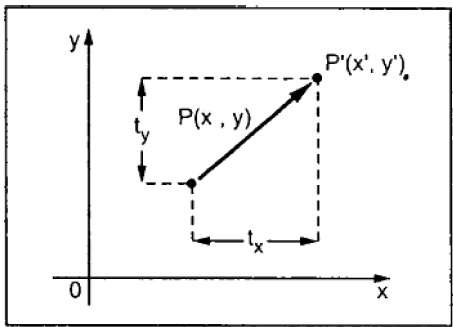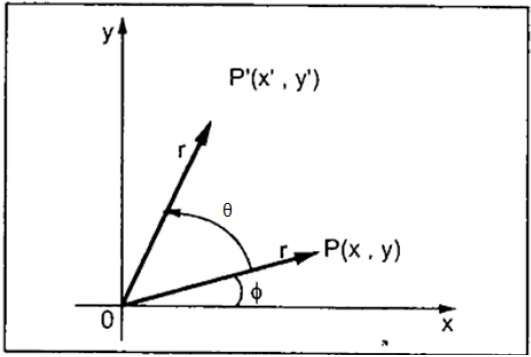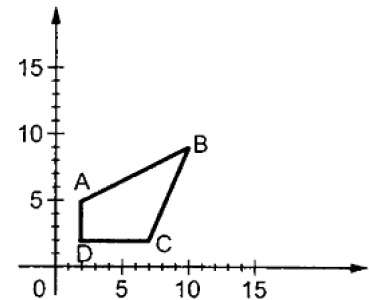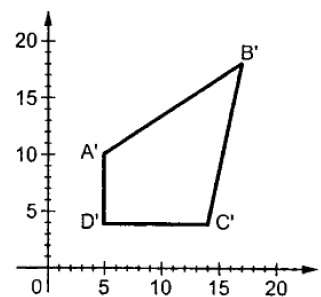# Matrix Representations and Homogeneous Coordinates

To perform a sequence of transformation such as translation followed by rotation and scaling, we need to follow a sequential process −

• Translate the coordinates,
• Rotate the translated coordinates, and then
• Scale the rotated coordinates to complete the composite transformation.

To shorten this process, we have to use 3×3 transformation matrix instead of 2×2 transformation matrix. To convert a 2×2 matrix to 3×3 matrix, we have to add an extra dummy coordinate W.

In this way, we can represent the point by 3 numbers instead of 2 numbers, which is called Homogeneous Coordinate system. In this system, we can represent all the transformation equations in matrix multiplication. Any Cartesian point P(X, Y) can be converted to homogeneous coordinates by P’ (Xh, Yh, h).

## Translation

A translation moves an object to a different position on the screen. You can translate a point in 2D by adding translation coordinate (tx, ty) to the original coordinate (X, Y) to get the new coordinate (X’, Y’).From the above figure, you can write that −

X’ = X + tx

Y’ = Y + ty

The pair (tx, ty) is called the translation vector or shift vector. The above equations can also be represented using the column vectors.

P=[X][Y]P=[X][Y] p’ = [X][Y][X′][Y′]T = [tx][ty][tx][ty]

We can write it as −

P’ = P + T

## Rotation

In rotation, we rotate the object at particular angle θ (theta) from its origin. From the following figure, we can see that the point P(X, Y) is located at angle φ from the horizontal X coordinate with distance r from the origin.

Let us suppose you want to rotate it at the angle θ. After rotating it to a new location, you will get a new point P’ (X’, Y’).Using standard trigonometric the original coordinate of point P(X, Y) can be represented as −

X=rcosϕ......(1)X=rcosϕ……(1)

Y=rsinϕ......(2)Y=rsinϕ……(2)

Same way we can represent the point P’ (X’, Y’) as −

x=rcos(ϕ+θ)=rcosϕcosθrsinϕsinθ.......(3)x′=rcos(ϕ+θ)=rcosϕcosθ−rsinϕsinθ…….(3)

y=rsin(ϕ+θ)=rcosϕsinθ+rsinϕcosθ.......(4)y′=rsin(ϕ+θ)=rcosϕsinθ+rsinϕcosθ…….(4)

Substituting equation (1) & (2) in (3) & (4) respectively, we will get

x=xcosθysinθx′=xcosθ−ysinθ

y=xsinθ+ycosθy′=xsinθ+ycosθ

Representing the above equation in matrix form,

[XY]=[XY][cosθsinθsinθcosθ]OR[X′Y′]=[XY][cosθsinθ−sinθcosθ]OR

P’ = P . R

Where R is the rotation matrix

R=[cosθsinθsinθcosθ]R=[cosθsinθ−sinθcosθ]

The rotation angle can be positive and negative.

For positive rotation angle, we can use the above rotation matrix. However, for negative angle rotation, the matrix will change as shown below −

R=[cos(θ)sin(θ)sin(θ)cos(θ)]R=[cos(−θ)sin(−θ)−sin(−θ)cos(−θ)]
=[cosθsinθsinθcosθ](cos(θ)=cosθandsin(θ)=sinθ)=[cosθ−sinθsinθcosθ](∵cos(−θ)=cosθandsin(−θ)=−sinθ)

## Scaling

To change the size of an object, scaling transformation is used. In the scaling process, you either expand or compress the dimensions of the object. Scaling can be achieved by multiplying the original coordinates of the object with the scaling factor to get the desired result.

Let us assume that the original coordinates are (X, Y), the scaling factors are (SX, SY), and the produced coordinates are (X’, Y’). This can be mathematically represented as shown below −

X’ = X . SX and Y’ = Y . SY

The scaling factor SX, SY scales the object in X and Y direction respectively. The above equations can also be represented in matrix form as below −

(XY)=(XY)[Sx00Sy](X′Y′)=(XY)[Sx00Sy]

OR

P’ = P . S

Where S is the scaling matrix. The scaling process is shown in the following figure.If we provide values less than 1 to the scaling factor S, then we can reduce the size of the object. If we provide values greater than 1, then we can increase the size of the object.